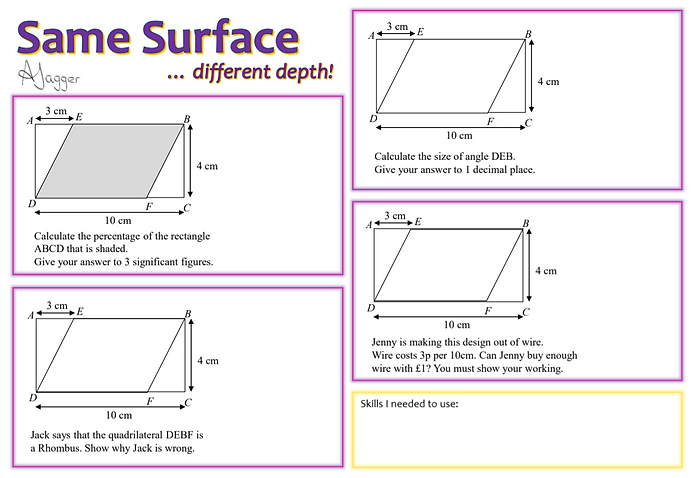Here are my same surface different depth problems!

Lots of students don’t read questions carefully enough and just assume what the question is asking them to do based on the basic information in the question.
These problems aim to combat this.

Each page contains 4 problems that, on the surface, appear the same (or at least very similar!)
However each question requires different skills to solve.
This means students have to read the question very carefully to deduce which skill they should be using.

Enjoy!

Skills required:

• Area of a rectangle

• Area of a parallelogram

• Calculate one number as a percentage of another

• Round to significant figures

Skills required:

• Trigonometry (SOHCAHTOA)

• Angles on  straight line add to 180                    or angles in parallel lines

• Rounding to decimal placesSkills required:

• Pythagoras' Theorem

• Reasoning

Skills required:

• Pythagoras' Theorem

• Perimeter

• Functional skills

• Reasoning